#### 期刊菜单

Study on Extraction Methods of Elevation Change Information Based on GaoFen-7
DOI: 10.12677/GST.2023.111004, PDF, HTML, XML, 下载: 21  浏览: 62

Abstract: To more accurately and timely grasp the terrain and terrain changes, this paper used the multi- phase GF7 stereo images to obtain the DSM of the same region at different time phases, respectively used the difference method and the ratio method to extract the elevation change information in this period, and compared the results obtained by the two extraction methods. The experiment results show that the difference method is more accurate and effective, and the accuracy of the change in-formation extraction is more than 80%. The extraction method is feasible and reliable, and it can timely grasp the terrain changes of the target area, which is of great help to the change discovery, update and maintenance of 3D Real Sense.

1. 引言

2. 数据准备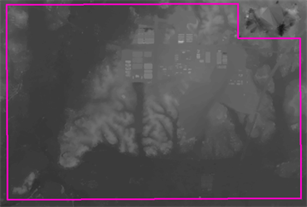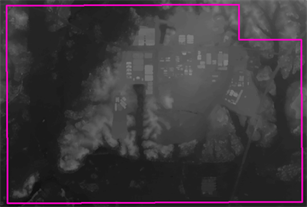(a) 研究区1 (平地) 2020年8月DSM (b) 研究区1 (平地) 2021年3月DSM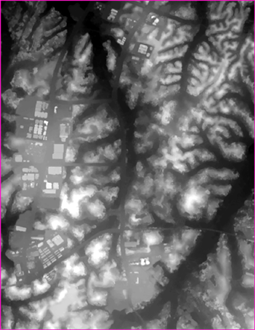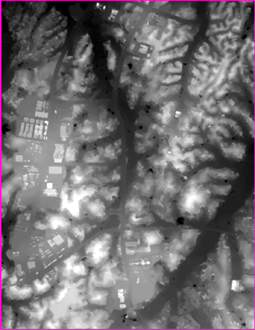(c) 研究区2 (丘陵地) 2021年3月DSM (d) 研究区2 (丘陵地) 2022年7月DSM

Figure 1. Different time phase of DSM in the study

3. 变化信息提取

3.1. 变化信息初步提取

$D{x}_{ij}^{k}={x}_{ij}^{k}\left({t}_{2}\right)-{x}_{ij}^{k}\left({t}_{1}\right)$ (1)

$D{x}_{ij}^{k}=\frac{{x}_{ij}^{k}\left({t}_{2}\right)}{{x}_{ij}^{k}\left({t}_{1}\right)}$ (2)

$D{x}_{ij}^{k}=\frac{{x}_{ij}^{k}\left({t}_{2}\right)+1}{{x}_{ij}^{k}\left({t}_{1}\right)+1}$ (3)

$D{x}_{ij}^{k}\le {T}_{1}$$D{x}_{ij}^{k}\le {T}_{h}$ (4)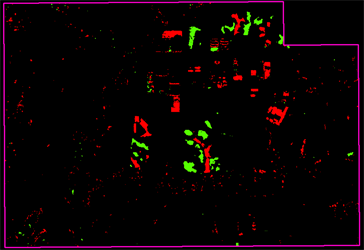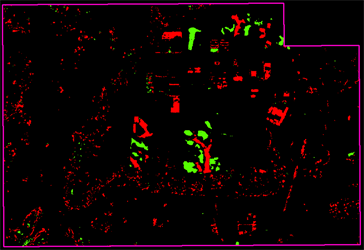(a) 差值法提取结果 (b) 比值法提取结果

Figure 2. Preliminary extraction results of elevation change information

3.2. 伪变化剔除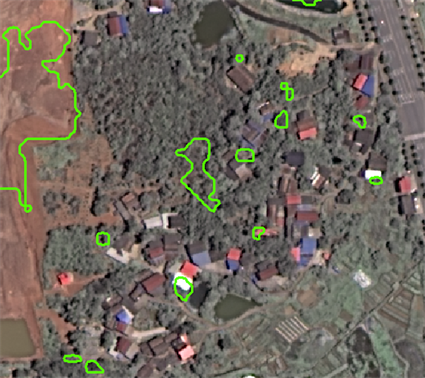Figure 3. Comparison between change extraction results and images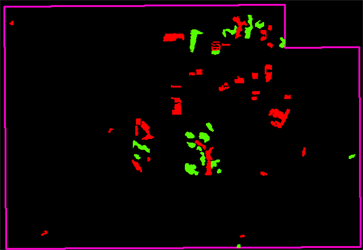Figure 4. Elevation change information after removing small area polygon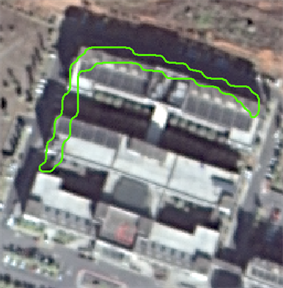Figure 5. Narrow pseudo change polygon

$a=\frac{{C}^{2}}{S}$ (5)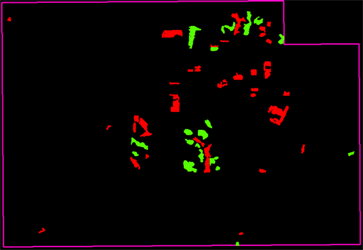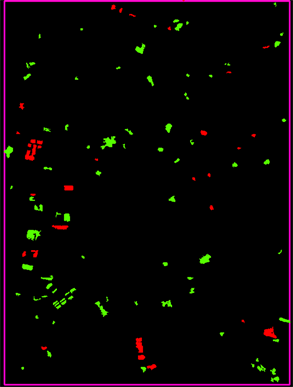(a) 研究区1 (平地) (b) 研究区2 (丘陵地)

Figure 6. Eliminate complex pseudo change polygon

3.3. 结果分析

$P=\frac{T}{T+F}×100%$ (6)Table 1. Date statistics of study 1 (flatland)Table 2. Date statistics of study 2 (hilly land)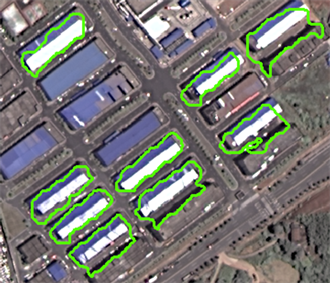Figure 7. Error polygon

4. 结束语

  张帆, 黄先锋, 高云龙, 等. 实景三维中国建设技术大纲(2021版)解读与思考[J]. 测绘地理信息, 2021(6): 171-174.  程小河, 段文华. 基于高分七号卫星影像的空三加密优化方案探索[J]. 地理空间信息, 2021(4): 1-3.  李国元, 唐新明, 陈继溢, 等. 高分七号卫星激光测高数据处理与精度初步验证[J]. 测绘学报, 2021(10): 1338-1348.  赵文普, 刘钰, 吴晓春, 等. 利用高分七号卫星开展1:10000立体测图精度验证[J]. 测绘科学, 2022(1): 165-171.  谢珍莲. 基于DSM自动提取变化信息的方法研究[J]. 经纬天地, 2021(2): 38-41.  范红娜. 基于DSM多尺度采样的建筑物变化检测与语义识别方法[D]: [硕士学位论文]. 新市: 辽宁工程技术大学, 2021.